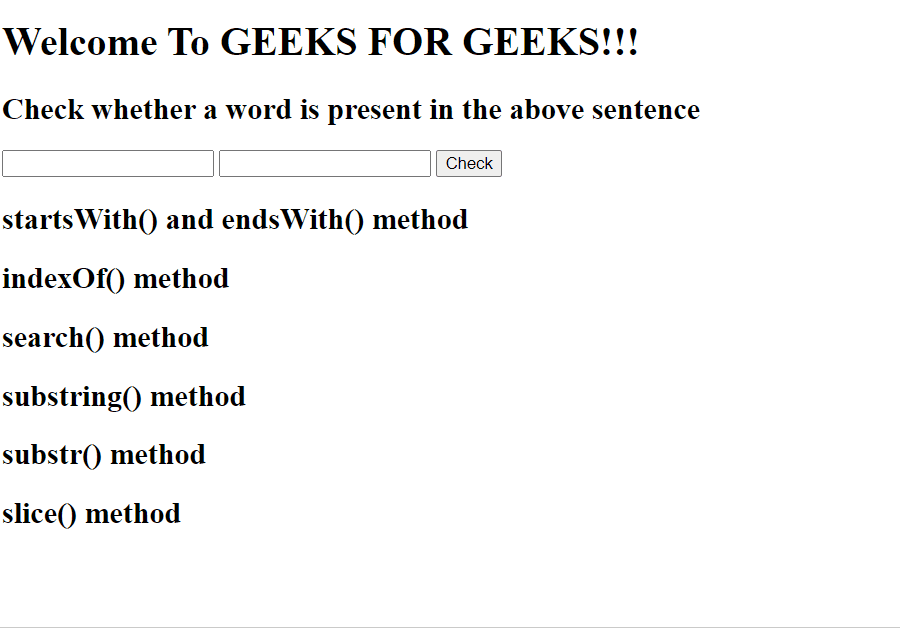GeeksforGeeks App
Open AppBrowser
Continue

# How to check a string starts/ends with a specific string in jQuery ?

JavaScript provides a lot of string methods that check whether a string is a substring of other string. So, jQuery wouldn’t be necessary at all to perform this task. However, we will cover all the different ways of checking whether a string starts or ends with a string:

Let’s consider a string str = “Welcome to GEEKS FOR GEEKS!!!”. Now we have to find whether the string str starts with startword = “Welcome” and ends with endword = “!!!”.

Approaches:

JavaScript startsWith() and endsWith() method: It checks whether a string starts or ends with the specific substring.

Syntax:

```if (str.startsWith(startword) && str.endsWith(endword)) {

// case sensitive
console.log("Yes, The string " + str + " starts with "
+ startword + " and ends with " + endword);
}```

JavaScript search() method: It checks whether a particular string is contained in another string and returns the starting index of that substring.

Syntax:

```if (str.search(startword)==0 &&
str.search(endword)==stringlength-endwordlength ) {

// case sensitive
console.log("Yes, The string " + str + " starts with "
+ startword + " and ends with " + endword);
}```

JavaScript indexOf() method: As the name suggests, it finds the starting index of a substring in the string.

Syntax:

```if (str.indexOf(startword)==0 &&
str.indexOf(endword)==stringlength-endwordlength) {

console.log("Yes, The string " + str + " starts with "
+ startword + " and ends with " + endword);
}```

JavaScript substring() method: It returns a string present between start and end index.

Syntax:

```if(str.substring(0, startwordlength)==startword
&& str.substring(stringlength-endwordlength,
stringlength)==endword) {

console.log("Yes, The string " + str + " starts with "
+ startword + " and ends with " + endword);
}```

JavaScript substr() method: It is similar to substring() method but takes start index and length of substring as parameters.

Syntax:

```if(str.substr(0, startwordlength)==startword
&& str.substr(stringlength-endwordlength,
endwordlength)==endword) {

console.log("Yes, The string " + str + " starts with "
+ startword + " and ends with " + endword);
}```

JavaScript slice() method: This method returns the slices of string between any two indices in a string.

Syntax:

```if(str.slice(0, startwordlength)==startword
&& str.slice(stringlength-endwordlength,
stringlength)==endword) {

console.log("Yes, The string " + str + " starts with "
+ startword + " and ends with " + endword);
}```

Let’s take an example of text in h1 element and check whether it starts and ends with the given substring.

## HTML

 ```<``html``>`` ` `<``head``>``    ``<``title``>``        ``How to know that a string starts/ends``        ``with a specific string in jQuery?``    ```` ` `    ``<``script` `src``=``"https://ajax.googleapis.com/ajax/libs/jquery/3.4.1/jquery.min.js"``>``    `````` ` `<``body``>``    ``<``h1` `id``=``"text"``>``        ``Welcome To GEEKS FOR GEEKS!!!``    ```` ` `    ``<``h2``>``        ``Check whether a word is present``        ``in the above sentence``    ```` ` `    ``<``input` `type``=``"text"` `id``=``"startkey"``>``    ``<``input` `type``=``"text"` `id``=``"endkey"``>``    ``<``button` `onclick``=``"MyFunction()"``> Check `` ` `    ``<``h2``> startsWith() and endsWith() method ``    ``<``h2` `id``=``"startswith"``> `` ` `    ``<``h2``> indexOf() method ``    ``<``h2` `id``=``"indexOf"``>`` ` `    ``<``h2``> search() method ``    ``<``h2` `id``=``"search"``>`` ` `    ``<``h2``> substring() method ``    ``<``h2` `id``=``"substring"``>`` ` `    ``<``h2``> substr() method ``    ``<``h2` `id``=``"substr"``> `` ` `    ``<``h2``> slice() method ``    ``<``h2` `id``=``"slice"``>`` ` ` ` `    ``<``script``>``        ``var str = document.getElementById("text").innerText;``        ``stringlength = str.length;``        ``var startword = "";``        ``var endword = "";``        ``function MyFunction() {``            ``startword = document.getElementById("startkey").value;``            ``endword = document.getElementById("endkey").value;``            ``console.log(startword);``            ``console.log(endword)``            ``startwordlength = startword.length;``            ``endwordlength = endword.length;`` ` `            ``if (str.startsWith(startword) && str.endsWith(endword)) {``                ``// case sensitive ``                ``var h2 = document.getElementById("startswith");``                ``h2.innerHTML =``                    ``"Yes, The string " + str + " starts with " ``                    ``+ startword + " and ends with " + endword;``            ``}``            ``else {``                ``var h2 = document.getElementById("startswith");``                ``h2.innerHTML =``                    ``"No, The string " + str + ``                    ``" doesn't start and endwith the given words";``            ``}``            ``//Js``            ``if (str.indexOf(startword) == 0 && str.indexOf(``                ``endword) == stringlength - endwordlength) {``                ``var h2 = document.getElementById("indexOf");``                ``h2.innerHTML =``                    ``"Yes, The string " + str + " starts with " ``                    ``+ startword + " and ends with " + endword;``            ``}``            ``else {``                ``var h2 = document.getElementById("indexOf");``                ``h2.innerHTML =``                    ``"No, The string " + str + ``                    ``" doesn't start and endwith the given words";``            ``}``            ``//Js``            ``if (str.search(``                ``startword) == 0 && str.search(endword)``                    ``== stringlength - endwordlength) {`` ` `                ``// case sensitive ``                ``var h2 = document.getElementById("search");``                ``h2.innerHTML = "Yes, The string " + str +``                        ``" starts with " + startword +``                        ``" and ends with " + endword;``            ``}``            ``else {``                ``var h2 = document.getElementById("search");``                ``h2.innerHTML = "No, The string " + str + ``                    ``" doesn't start and endwith the given words";``            ``}``            ``//Js``            ``if (str.substring(``                ``0, startwordlength) == startword && str.substring(``                    ``stringlength - endwordlength, stringlength) == endword) {``                ``var h2 = document.getElementById("substring");``                ``h2.innerHTML =``                    ``"Yes, The string " + str + " starts with " ``                    ``+ startword + " and ends with " + endword;``            ``}``            ``else {``                ``var h2 = document.getElementById("substring");``                ``h2.innerHTML =``                    ``"No, The string " + str + ``                    ``" doesn't start and endwith the given words";``            ``}`` ` `            ``if (str.substr(``                ``0, startwordlength) == startword && str.substr(``                    ``stringlength - endwordlength, endwordlength) == endword) {``                ``var h2 = document.getElementById("substr");``                ``h2.innerHTML =``                    ``"Yes, The string " + str + " starts with " ``                    ``+ startword + " and ends with " + endword;``            ``}``            ``else {``                ``var h2 = document.getElementById("substr");``                ``h2.innerHTML =``                    ``"No, The string " + str + ``                    ``" doesn't start and endwith the given words";``            ``}``            ``if (str.slice(``                ``0, startwordlength) == startword && str.slice(``                    ``stringlength - endwordlength, stringlength) == endword) {``                ``var h2 = document.getElementById("slice");``                ``h2.innerHTML =``                    ``"Yes, The string " + str + " starts with " + ``                    ``startword + " and ends with " + endword;``            ``}``            ``else {``                ``var h2 = document.getElementById("slice");``                ``h2.innerHTML =``                    ``"No, The string " + str + ``                    ``" doesn't start and endwith the given words";``            ``}``        ``}``    `````` ` ``

Output:How to check a string starts/ends with a specific string in jQuery ?

My Personal Notes arrow_drop_up
Related Tutorials